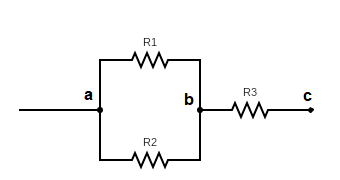# Maximum voltage that can be applied to DC circuit with resistors

• Sokolov

#### Sokolov

Homework Statement
In a DC circuit, two resistors ##R_1## and ##R_2## are connected in parallel, and a third resistor ##R_3## is connected in series with them, as shown in the picture. If the maximum power that each of these 3 resistors can hold is ##P_{max}##, which would be the maximum voltage that could be applied between the points a and b?
Relevant Equations
Kirchhoff's laws: ##I_1+I_2=I_3##
The power dissipated by a resistor: ##P=I^2R=IV=V^2/R##I see that ##V_{ac}=V_{ab}+V_{bc}##, with ##V_{ab}=I_1R_1=I_2R_2## and ##V_{bc}=I_3R_3##.

how to express mathematically the maximum value of ##V_{ac}##
Can you do it with ##V_{ab}## or ##V_{bc}## ?

Can you do it with ##V_{ab}## or ##V_{bc}## ?
I think that the result must be given in terms of ##P_{max}##. The exercise ask also to apply the result for the particular case that ##R1=2.8 kΩ, R2=3.7 kΩ, R3=1.8 kΩ## and ##P_{max}=0.5W##

I agree, but you must start somewhere. Use ##P_{\text {max}} ## and ##R_i## (as symbols).
How many equations witth how many unknowns do yuo have ? Do you have to distinguish cases ?

If the parallel circuit is annoying, use the equivalent resistance.